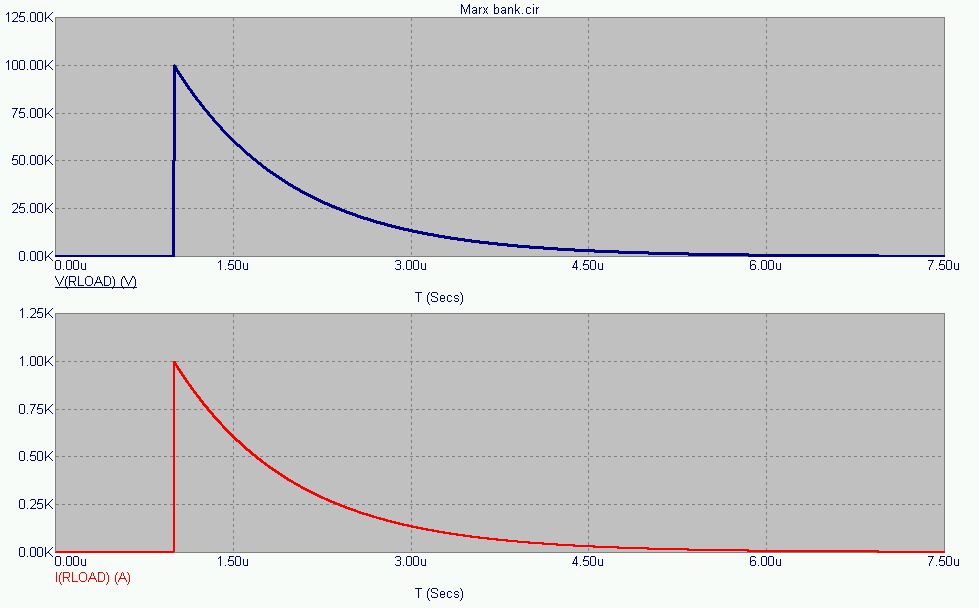NESS ENGINEERING
TECHNICAL DATA
MARX GENERATORS

Marx Generators are often used in pulsed power and high voltage applications to generate a high peak voltage pulse. The simplified theory behind them is that a number of capacitors are charged in parallel and then switches are closed to connect them in series with the charge voltage adding up so that the output voltage is approximately equal to NVo with N being the number of Marx stages and Vo being the initial charging voltage.

In low rep-rate applications, resistors are used as charging isolation components. In high rep-rate applications, the power dissipation within these elements becomes unacceptably large and inductors must be utilized.

Each of the following waveform plots can be clicked on to open up the full size graph in a separate window.

The circuit schematic for a 10 stage Marx generator is shown below. In this specific model, each stage capacitor is 100nF and is charged to 10 kV. At 1 ms into the simulation, each of the stage switches close and the Marx erects, connecting each capacitor in series. The equivalent series capacitance (10nF) then discharges into the load resistor with a peak voltage of 100 kV.The results of the circuit model are shown below. V(RLOAD) is the voltage on the 100 ohm load resistor after the Marx fully erects. The circuit current, I(RLOAD) is graphed in the second, lower plot.Omega L is the circuit inductance (H)  C is the circuit capacitance (F)  R is the circuit resistance (W) Current V0 is the initial voltage on the capacitance (V) Peak Current Same as above

##### Send consulting inquiries, comments, and suggestions to nessengr@san.rr.com

Ness Engineering, Inc.
P.O. Box 261501
San Diego, CA 92196
(858) 566-2372
(858) 240-2299 FAX

 © Richard M. Ness and Ness Engineering, Inc. Last Updated: